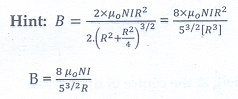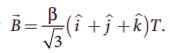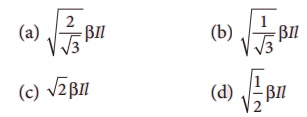Home | | Physics 12th Std | Magnetism and Magnetic Effects of Electric Current: Multiple choice questions

# Magnetism and Magnetic Effects of Electric Current: Multiple choice questions

Physics : Magnetism and Magnetic Effects of Electric Current: Multiple choice questions with Answers, Solution and Explanation

Multiple choice questions

1.The magnetic field at the center O of the following current loop isAns: (a)2. An electron moves straight inside a charged parallel plate capacitor of uniform charge density σ. The time taken by the electron to cross the parallel plate capacitor when the plates of the capacitor are kept under constant magnetic field of inductionisAns: (d)3.The force experienced by a particle having mass m and charge q accelerated through a potential difference V when it is kept under perpendicular magnetic fieldisAns: (c)4.A circular coil of radius 5 cm and 50 turns carries a current of 3 ampere. The magnetic dipole moment of the coil is

(a) 1.0 amp – m2

(b) 1.2 amp – m2

(c) 0.5 amp – m2

(d) 0.8 amp – m25. A thin insulated wire forms a plane spiral of N = 100 tight turns carrying a current I = 8 m A (milli ampere). The radii of inside and outside turns are a = 50 mm and b = 100 mm respectively. The magnetic induction at the center of the spiral is

(a) 5 µT

(b) 7 µT

(c) 8 µT

(d) 10 µT6. Three wires of equal lengths are bent in the form of loops. One of the loops is circle, another is a semi-circle and the third one is a square. They are placed in a uniform magnetic field and same electric current is passed through them. Which of the following loop configuration will experience greater torque ?

(a) circle

(b) semi-circle

(c) square

(d) all of them7.Two identical coils, each with N turns and radius R are placed coaxially at a distance R as shown in the figure. If I is the current passing through the loops in the same direction, then the magnetic field at a point P which is at exactly at R/2 distance between two coils isAns: (b)8. A wire of length l carries a current I along the Y direction and magnetic field is given byThe magnitude of Lorentz force acting on the wire isAns: (a)9. A bar magnet of length l and magnetic moment M is bent in the form of an arc as shown in figure. The new magnetic dipole moment will bea.     M

b.    3/ π M

c.      2/ π M

d.     ½ M10. A non-conducting charged ring of charge q, mass m and radius r is rotated with constant angular speed ω. Find the ratio of its magnetic moment with angular momentum is

a.     q/m

b.     2q/m

c.      q/2m

d.     q/4m11. The BH curve for a ferromagnetic material is shown in the figure. The material is placed inside a long solenoid which contains 1000 turns/ cm. The current that should be passed in the solenonid to demagnetize the ferromagnet completely is(a) 1.00 m A (milli ampere)

(b) 1.25 mA

(c) 1.50 mA

(d) 1.75 mA12.  Two short bar magnets have magnetic moments  1.20  Am2  and  1.00  Am2 respectively. They are kept on a horizontal table parallel to each other with their north poles pointing towards the south. They have a common magnetic equator and are separated by a distance of 20.0 cm. The value of the resultant horizontal magnetic induction at the mid-point O of the line joining their centers is (Horizontal components of Earth’s magnetic induction is 3.6 × 10-5 Wb m-2 )

a.     3.60 × 10-5 Wb m-2

b.     3.5 × 10-5 Wb m-2

c.      2.56 × 10-4 Wb m-2

d.     2.2 × 10-4 Wb m-213. The vertical component of Earth’s magnetic field at a place is equal to the horizontal component. What is the value of angle of dip at this place?

(a) 30º

(b) 45 º

(c) 60 º

(d) 90 º14. A flat dielectric disc of radius R carries an excess charge on its surface. The surface charge density is σ. The disc rotates about an axis perpendicular toits plane passing through the center with angular velocity ω. Find the magnitude of the torque on the disc if it is placed in a uniform magnetic field whose strength is B which is directed perpendicular to the axis of rotationAns: (d)15. A simple pendulum with charged bob is oscillating with time period T and let θ be the angular displacement. If the uniform magnetic field is switched ON in a direction perpendicular to the plane of oscillation then

a.     time period will decrease but θ will remain constant

b.     time period remain constant but θ will decrease

c.      both T and θ will remain the same

d.     both T and θ will decrease

Hint: Both T θ and decreases as B increases.

Tags : Physics , 12th Physics : Magnetism and Magnetic Effects of Electric Current
Study Material, Lecturing Notes, Assignment, Reference, Wiki description explanation, brief detail
12th Physics : Magnetism and Magnetic Effects of Electric Current : Magnetism and Magnetic Effects of Electric Current: Multiple choice questions | Physics